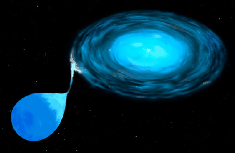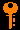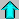# Lecture 6 - Spectroscopic and Eclipsing Binaries (1/28/99)Binaries I --- | --- H-R DiagramChapter 12 (ZG4)

Notes:

pages 20 - 23

Artists conception of an X-ray binary star. The two stars are so close together that the larger star spills over onto the white dwarf companion, which gets heated and emits X-rays. (Courtesy NASA/STSCI)
Key Question:## Investigations:

1. Spectroscopic Binaries
• What is a Dopper shift?
• How do you find radial velocity from the observed wavelengths of spectral lines?
• What is the radial velocity corresponding to a 1 Angstrom shift at 5000 Angstroms rest wavelength?
• What is the shift corresponding to a 1 km/s radial velocity at 5000 Angstroms?
• What is the resolving power of a high resolution spectrograph needed to measure radial velocities with < 1 km/s accuracy?
• What is the orbital velocity of the Earth around the Sun?
• What is the orbital velocity of the Sun induced by Jupiter?
• What is a double-line spectroscopic binary?
• What role does the inclination of the orbit play in the observed velocities?
• How do you find the masses from a double-line binary?
• What is a single-line spectroscopic binary?
• What is the mass function for a binary star?
• How do you find the mass function of a single line binary?
• What is the significance of the mass function, given that the total mass and the inclination are unknown?
• What effect do orbital eccentricity and inclination have on the observed velocity curves?
2. Eclipsing Binaries
• What is the likely inclination and/or separation of an eclipsing binary system?
• How do you recognize an eclipsing binary system?
• What are the times of 1st - 4th contact?
• How do you find the radii of the stars from the light curves?
• How are the depths of the eclipses related to the temperatures of the stars? Why?
• If the eclipsing binary is also spectroscopic, what additional information can be learned?
3. Example (see homework 1): Sirius A & B - Radii and Densities
• System Data II -

 Bolometric Magnitude (Sirius A): +1.33 Bolometric Magnitude (Sirius B): +8.57 Bolometric Magnitude (Sun): +4.76 Effective Temperature (Sirius A) 9200 K Effective Temperature (Sirius B) 27000 K Effective Temperature (Sun) 5770 K

• What are the luminosities of Sirius A & B in Lsun?
• What are the radii of Sirius A & B?
• What are the mean densities of Sirius A & B?
• Where on the H-R diagram do Sirius A & B lie?
• What stellar classifications would you give to Sirius A & B?

## The Doppler Effect

The Doppler effect:Prev Lecture ---Next Lecture ---Astr12 Index ---Astr12 Home

smyers@nrao.edu Steven T. Myers